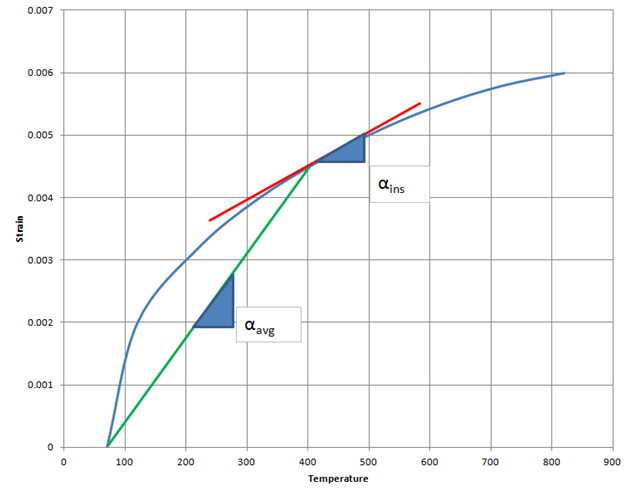you are here:/ News & Insights / Engineering Advantage Blog / Using Coefficients of Thermal Expansion in a Finite Element Model

# Using Coefficients of Thermal Expansion in a Finite Element ModelOctober 31, 2014 By: James Kosloski

When using a coefficient of thermal expansion in a finite element model, you must be careful when dealing with temperature dependant data.  There are generally 2 ways of specifying coefficient of thermal expansion: Instantaneous (tangent) or Average (secant).  Understanding the difference between these two definitions of CTE is critical.If we look at a plot of thermal strain vs. temperature, the average CTE is a measure of the thermal strain generated due a change in temperature from a specific reference temperature to the current temperature.  The instantaneous CTE represents the thermal strain generated due to an infinitesimal change in temperature around the current temperature.

You can see that average CTEs generated using 1 reference temperature cannot be used with a different reference temperature. For example, if I know the thermal strain generated due to heating a part up from 70 degrees to 400 degrees, I cannot use that same CTE to calculate the thermal strain generated in a cool down from 730 degrees to 400 degrees, even though the final temperature and the delta temperature are the same.

You MUST make sure that any average temperature dependant CTE’s entered are corrected for the operating reference temperature. Many FEA codes can do this conversion automatically, for example, the MPAMOD command in ANSYS will convert average CTE from one reference temperature to another.

If you have instantaneous data instead of average data, there is no conversion needed. Instantaneous data is valid for any reference temperature.

So, be sure to understand what your CTE data represents before using it in a finite element model!